#### How to Use COUNTIFS Function

The COUNTIFS function in Excel is a powerful tool that allows you to count the number of cells in a range that meet multiple conditions. This function is useful for analyzing data, finding patterns, and making decisions based on specific criteria.

In this Excel tutorial article, we will try to learn all the possible ways to use the COUNTIFS functions.

## Countifs function basics

Let us start with a simple COUNTIFS function. Let us use simple data and use the formula: =COUNTIFS(A1:A6,”4″)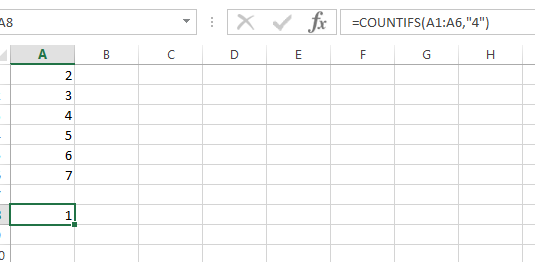The result is 1, as it found a simple instance of 4.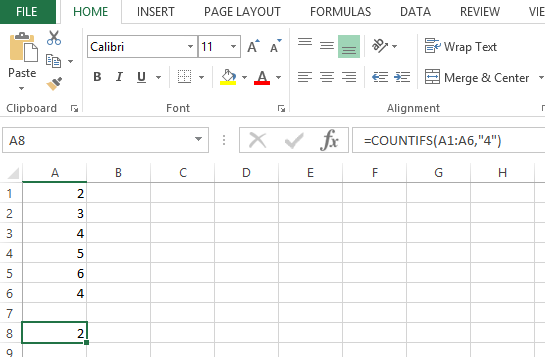But if we have more than one condition in more than one range, we will have to use the COUNTIFS instead of the simple countif excel function.

Let’s have the following data. Find someone from team1 with 4 marks: =COUNTIFS(A2:A7,A4,B2:B7,B2)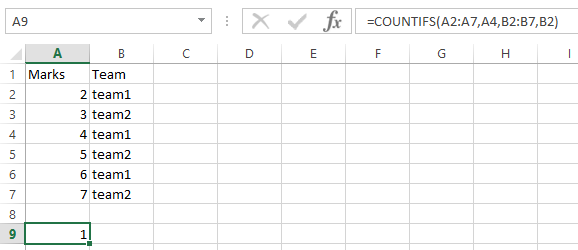See if we have more conditions. I’m adding more data.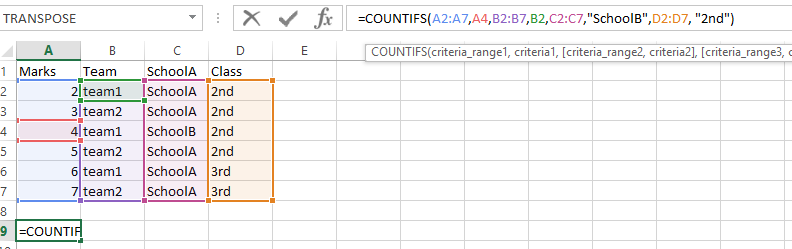Let us see the result of the following formula: =COUNTIFS(A2:A7,A4,B2:B7,B2,C2:C7,”SchoolB”,D2:D7, “2nd”)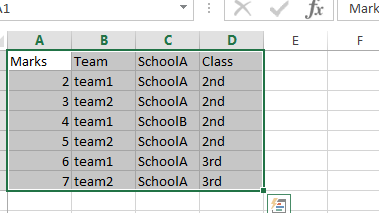Please find below the result: 1 (row 4).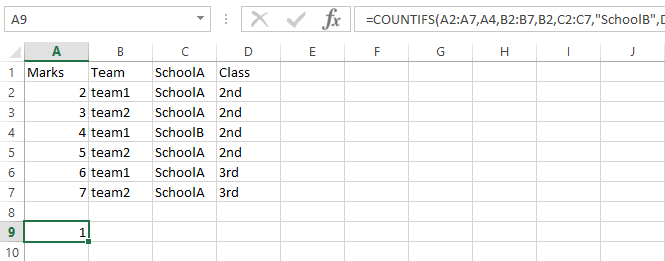Note: The COUNTIFS function can be used with up to 127 ranges and criteria. If you need to count cells with more than 127 criteria, you can use the SUMPRODUCT function in combination with the COUNTIF function.

In conclusion, the COUNTIFS function in Excel is a useful tool for counting the number of cells in a range that meet multiple conditions. With the COUNTIFS function, you can easily analyze data, find patterns, and make decisions based on specific criteria. Whether you’re working with sales data, inventory data, or any other type of data, the COUNTIFS function can help you get the insights you need to make informed decisions.